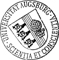## Berry-Esseen Bounds and Cramer-Type Large Deviations for the Volume Distribution of Poisson Cylinder Processes

• A stationary Poisson cylinder process is composed by a stationary Poisson process of k-dimensional affine subspaces in a d-dimensional Euclidean space (1 <= k < d) which are dilated by a family of independent identically distributed random compact cylinder bases taken from the corresponding orthogonal complement. We study the accuracy of normal approximation of the d-volume of the union set of Poisson cylinders that covers cW when the scaling factor c > 0 becomes large. Here W denotes some fixed compact star-shaped window set containing the origin as inner point. We give lower and upper bounds of the variance of the union set in cW which exhibit long-range dependence within the union set of cylinders. Our main results are sharp estimates of the higher-order cumulants of the d-volume of all Poisson cylinders in cW under the assumption that the (d-k)-volume of the typical cylinder base possesses a finite exponential moment. These estimates enable us to apply the celebrated Lemma on largeA stationary Poisson cylinder process is composed by a stationary Poisson process of k-dimensional affine subspaces in a d-dimensional Euclidean space (1 <= k < d) which are dilated by a family of independent identically distributed random compact cylinder bases taken from the corresponding orthogonal complement. We study the accuracy of normal approximation of the d-volume of the union set of Poisson cylinders that covers cW when the scaling factor c > 0 becomes large. Here W denotes some fixed compact star-shaped window set containing the origin as inner point. We give lower and upper bounds of the variance of the union set in cW which exhibit long-range dependence within the union set of cylinders. Our main results are sharp estimates of the higher-order cumulants of the d-volume of all Poisson cylinders in cW under the assumption that the (d-k)-volume of the typical cylinder base possesses a finite exponential moment. These estimates enable us to apply the celebrated Lemma on large deviations' due to V. Statulevicius.• Dokument_1.pdfAuthor: Lothar HeinrichGND, Malte Spiess urn:nbn:de:bvb:384-opus4-11043 https://opus.bibliothek.uni-augsburg.de/opus4/1311 Preprints des Instituts für Mathematik der Universität Augsburg (2009-26) Preprint English Universität Augsburg Universität Ulm, Institut für Stochastik 2009/10/16 stationary random sets; stochastic geometry; central limit theorem; higher-order (mixed) cumulant; moment- and cumulant generating function Stochastische Geometrie; Poisson-Prozess; Zentraler Grenzwertsatz; Große Abweichung; Asymptotische Approximation Mathematisch-Naturwissenschaftlich-Technische Fakultät Mathematisch-Naturwissenschaftlich-Technische Fakultät / Institut für Mathematik Mathematisch-Naturwissenschaftlich-Technische Fakultät / Institut für Mathematik / Lehrstuhl für Stochastik und ihre Anwendungen 5 Naturwissenschaften und Mathematik / 51 Mathematik / 510 MathematikDeutsches Urheberrecht mit Print on Demand